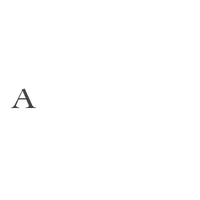Revel in the opulence of our glorious traditions with our HERITAGE collection – a timeless ode to the unforgettable jewels of royal India. Colorful, vibrant and set in rich tones of gold with diamonds, our festive range captures the spirit of Indian celebrations.

Rs. 500,000

Rs. 340,000

Rs. 590,000

Rs. 490,000

Rs. 310,000

Rs. 470,000

Rs. 694,000

Rs. 281,000

Rs. 886,000

Rs. 190,000

Rs. 310,000

Rs. 1,245,000

Rs. 721,000

Rs. 470,000

Rs. 270,000

Rs. 1,035,000

Rs. 423,000

Rs. 1,300,000

Rs. 225,000

Rs. 950,000

Rs. 2,000,000

Rs. 392,000

Rs. 423,000

Rs. 580,000

Rs. 2,050,000

Rs. 590,000

Rs. 753,000

Rs. 884,000

Rs. 2,630,000

Rs. 105,000

Rs. 555,000

Rs. 430,000

Rs. 110,000

Rs. 600,000

Rs. 2,300,000

Rs. 341,000

Rs. 630,000

Rs. 264,000

Rs. 460,000

Rs. 210,000

Rs. 256,000

Rs. 330,000

Rs. 90,000

Rs. 860,000

Rs. 1,700,000

Rs. 226,000

Rs. 3,000,000

Rs. 190,000

Rs. 400,000

Rs. 158,000

Rs. 310,000

Rs. 650,000

Rs. 385,000

Rs. 1,008,000

Rs. 430,000

Rs. 1,000,000

Rs. 1,072,000

Rs. 1,250,000

Rs. 235,000

Rs. 325,000

Rs. 350,000

Rs. 318,000

Rs. 475,000

Rs. 190,000

Rs. 318,000

Rs. 860,000

Rs. 532,000

Rs. 582,000

Rs. 870,000

Rs. 670,000

Rs. 600,000

Rs. 475,000

Rs. 1,082,000

Rs. 1,831,000

Rs. 1,400,000

Rs. 1,150,000

Rs. 460,000

Rs. 198,000

Rs. 580,000

Rs. 308,000

Rs. 392,000

Rs. 215,000

Rs. 187,000

Rs. 471,000

Rs. 492,000

Rs. 400,000

Rs. 260,000

Rs. 350,000

Rs. 345,000

Rs. 366,000

Rs. 150,000

Rs. 137,000

Rs. 136,000

Rs. 300,000